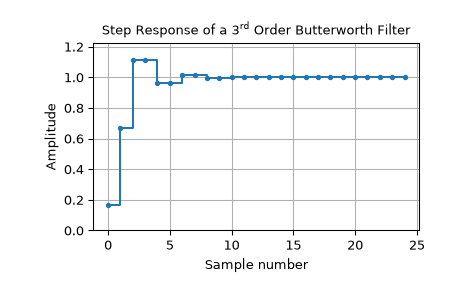# scipy.signal.dstep¶

scipy.signal.dstep(system, x0=None, t=None, n=None)[source]

Step response of discrete-time system.

Parameters
systemtuple of array_like

A tuple describing the system. The following gives the number of elements in the tuple and the interpretation:

• 1: (instance of `dlti`)

• 3: (num, den, dt)

• 4: (zeros, poles, gain, dt)

• 5: (A, B, C, D, dt)

x0array_like, optional

Initial state-vector. Defaults to zero.

tarray_like, optional

Time points. Computed if not given.

nint, optional

The number of time points to compute (if t is not given).

Returns
toutndarray

Output time points, as a 1-D array.

youttuple of ndarray

Step response of system. Each element of the tuple represents the output of the system based on a step response to each input.

Examples

```>>> from scipy import signal
>>> import matplotlib.pyplot as plt
```
```>>> butter = signal.dlti(*signal.butter(3, 0.5))
>>> t, y = signal.dstep(butter, n=25)
>>> plt.step(t, np.squeeze(y))
>>> plt.grid()
>>> plt.xlabel('n [samples]')
>>> plt.ylabel('Amplitude')
```